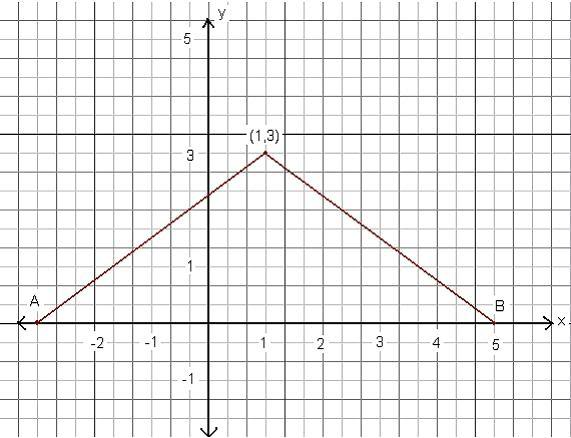# Describe a locus Algebraically

• jvercetti

## Homework Statement

Describe the locus algebraically. Then graph the locus and describe the locus geometrically. Prove that the locus is the perpendicular bisector of the line joining the two points.
Locus of points equidistant from (0,0) and (-6, -6)

## Homework Equations

None that I can think of

## The Attempt at a Solution

Here's what I have done so far:
Locus of points equidistant from (-3,0) and (5,0)
Geometric description: P(x,y) where PA= PB where A = (-3,0) and B is always isosceles.
The locus is the perpendicular bisector of line AB. This occurs because the locus crosses line AB at its midpoint.
Algebraic description: Confused Here :shy:
Graph:Graphically - I cannot see the locus.
Geometrically - B is isoceles? what is?
You have not yet shown the locus is a straight line, or that it is perpendicular to AB, in fact you have shown it at all.

Algebraically - what is the equation of the locus

?? In the problem statement you say "Locus of points equidistant from (0,0) and (-6, -6)" but in attempt at a solution you say "Locus of points equidistant from (-3,0) and (5,0)". Which is it?

In either case, you are correct that, geometrically, the locus is the perpendicular bisector of the line segment having those endpoints.

The "algebraic description" means the equation of that line. What are the coordinates of the midpoint of the given line segement? What is the slope of the line through the given points? What is the slope of the line perpendicular to that?

Finally, your graph shows (-3, 0) and (5,0) (but not (0,0) and (-6, -6)) and shows one example of a point on the locus but does not show the locus which is what you are asked to graph.

"The locus is the perpendicular bisector of line AB"

Actually, that should be "the locus is the perpendicular bisector of line segment AB." So, you just need the equation of the perpendicular bisector of that segment.

Ok sorry.. I found the mistake I was making with the question.. Sorry about typing the question wrong.. I mixed two questions up.. Anyways the question I had, I found the answer to.. Sorry for the trouble